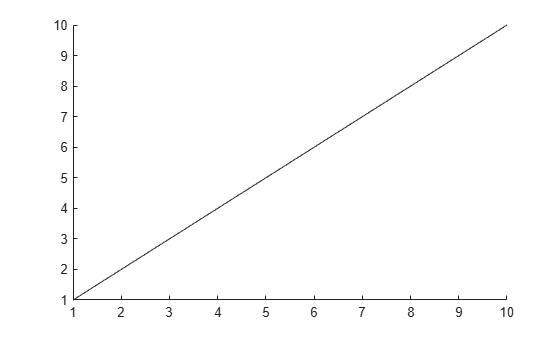# getpoints

## 语法

``[x,y] = getpoints(an)``
``[x,y,z] = getpoints(an) ``

## 说明

``[x,y] = getpoints(an)` 返回用于定义 `an` 所指定动画线条的点的 x 和 y 坐标。使用 `animatedline` 函数创建一根动画线条。`
``[x,y,z] = getpoints(an) ` 返回由 `an` 指定的三维动画线条的坐标。如果线条没有任何 z 值，则 `getpoints` 将 `z` 作为长度与 `x` 和 `y` 相同的零向量返回。`

## 示例

`h = animatedline(1:10,1:10);``[x,y] = getpoints(h)`
```x = 1×10 1 2 3 4 5 6 7 8 9 10 ```
```y = 1×10 1 2 3 4 5 6 7 8 9 10 ```

## 输入参数

`AnimatedLine` 对象。使用 `animatedline` 函数创建一个 `AnimatedLine` 对象。